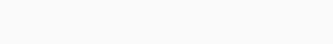# Left rotate an array by D places in Java

Hi, in this tutorial you will learn how to left rotate an Array by D places in JAVA.

Before beginning it is better if you are already familiar with the concept of arrays.As we can see in the above example we have an input array with the shift distance(D) as 4.

After rotation, the array would appear as:### Function For Rotation

```    public static int[] leftRotate(int array[], int d) {
d = d % array.length;
if (d == 0) {
return array;
}

int[] retArray2 = new int[array.length];
for (int i = 0; i < array.length; i++) {
if (i - d < 0) {
retArray2[array.length - (d - i)] = array[i];
} else {
retArray2[i - d] = array[i];
}
}
return retArray2;
}```

#### Algorithm Explanation

`d = d % array.length;`

This expression is used as if the shift distance is greater than the array length which makes the rotation complete once or more and then initiate it again. So it is better to bring the shift distance value to a value smaller than the array length for better efficiency.

```    if (d == 0) {
return array;
}```

This is if the shift distance becomes zero after decreasing the value and hence iterating through the array is not needed.

```int[] retArray = new int[array.length];
for (int i = 0; i < array.length; i++) {
if (i - d < 0) {
retArray[array.length - (d - i)] = array[i];
} else {
retArray[i - d] = array[i];
}
}```

First, we initialize a new array to store the rotated array.

Then, we begin to iterate through the array and start storing the value at index [I] on index [i – d].

In case, [i – d] is less than 0 we store the value of index [i] on index [array_length – d + i].

When the loop is finished the retArray would be the rotated array we wanted and will be returned.

### Main Driver Function

```   public static void main(String[] args) {
int array[] = {1, 2, 3, 4, 5, 6, 7, 8, 9, 10};
System.out.println("Original Array \n");
for (int i = 0; i < array.length; i++) {
System.out.print(array[i] + " ");
}
int rotatedArray[] = leftRotate(array, 4);

System.out.println("\n\nRotated Array \n");
for (int i = 0; i < array.length; i++) {
System.out.print(rotatedArray[i] + " ");
}
}```

This would be the main driver function from which the left rotating function would be called.

### Output

After running the above code we would get the following output :

```Original Array

1 2 3 4 5 6 7 8 9 10

Rotated Array

5 6 7 8 9 10 1 2 3 4```